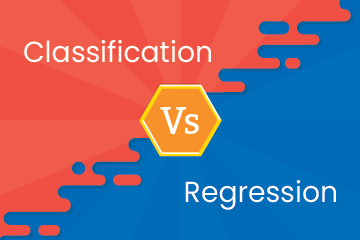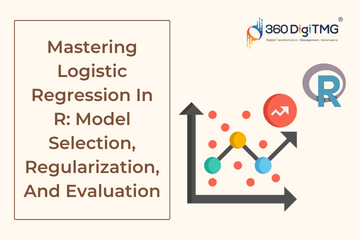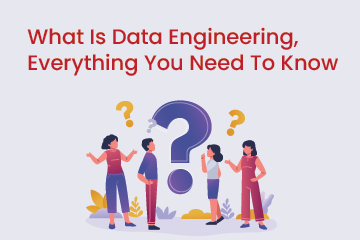Home / Blog / Data Science / Classification vs Regression

# Classification vs Regression

• March 14, 2023
• 2860
• 99### Meet the Author : Mr. Bharani Kumar

Bharani Kumar Depuru is a well known IT personality from Hyderabad. He is the Founder and Director of Innodatatics Pvt Ltd and 360DigiTMG. Bharani Kumar is an IIT and ISB alumni with more than 17 years of experience, he held prominent positions in the IT elites like HSBC, ITC Infotech, Infosys, and Deloitte. He is a prevalent IT consultant specializing in Industrial Revolution 4.0 implementation, Data Analytics practice setup, Artificial Intelligence, Big Data Analytics, Industrial IoT, Business Intelligence and Business Management. Bharani Kumar is also the chief trainer at 360DigiTMG with more than Ten years of experience and has been making the IT transition journey easy for his students. 360DigiTMG is at the forefront of delivering quality education, thereby bridging the gap between academia and industry.

Predictive Analytics models are widely used in Machine Learning and AI. And the two types of predictive analytics that are widely used in supervised Machine Learning are classification models and regression models. While classification models discrete variables, regression models continuous variables. Comparing classification to regression confuses the senior Data Scientists too, making it difficult for the new data scientists to implement the right methodology to solve the problems. They are both supervised learning models and are trained with existing data, but there are a lot of differences between them. Implementing the correct methodology gives out the answer you are looking for.

Want to learn more about data science? Enroll in this best data science course in Bangalore with placement to do so.

### Classification Analytics Model

As the name suggests Classification analytics model identifies a class or a category among various categories. In clearer words, it identifies a class among a set of variables that are already established and the algorithm will be able to recognize the new variable and where it belongs.

Classification models are hugely used to make binary predictions and the algorithm predicts two options and which is likely to happen, but it will function only when examples are classified into two or more classes.

### The different types of classification algorithms include

Decision Trees

Decision Trees are applicable in both classification and regression methods. It is built by building a decision tree where every node of the tree represents a test result and respective branches are possibles values of the attribute.

Random Forests

A Random Forest is a group of decision tree results that are increased into one final result. They can limit overfitting without increasing errors caused by bias; this makes them strong models. By training on different samples of data, Random Forests can reduce variance.

K-Nearest Neighbour

KNN algorithmMachine Learning is one of the uncomplicated ML algorithms based on the Supervised Learning Technique. It assumes the similarities between the new and available data and categorizes them based on pre-existing groups while storing all the available data. KNN incorporates a data point based on similarities of new and old data.

### Regression Analytics Model

Regressions models are complex and predict the performance of a process, product, or professional. The Regression models will give a result of what will happen from an infinite number of possibilities.

Become a Data Scientist with 360DigiTMG Data Scientist course in Hyderabad. Get trained by the alumni from IIT, IIM, and ISB.

### The different types of Regression algorithms include:

Simple Linear Regression

With the help of simple linear regression, you can estimate the relationship between an independent and a dependent variable using a straight line if both variables are quantitative.

Multiple linear regression

It is an extension of simple linear regression, it can predict the value of dependent variables with the help of multiple independent variables.

Polynomial Regression

It is mainly used to find a nonlinear relationship between the independent ad dependent variables.

### Classification VS Regression in Machine Learning

The important difference between regression and classification is while the former predicts continuous quality, the latter predicts discrete class labels. The classification can predict a continuous value in the form of class label probability, while regression predicts a discrete value in the form of an integer.

### Data Analyst Courses in Other Locations